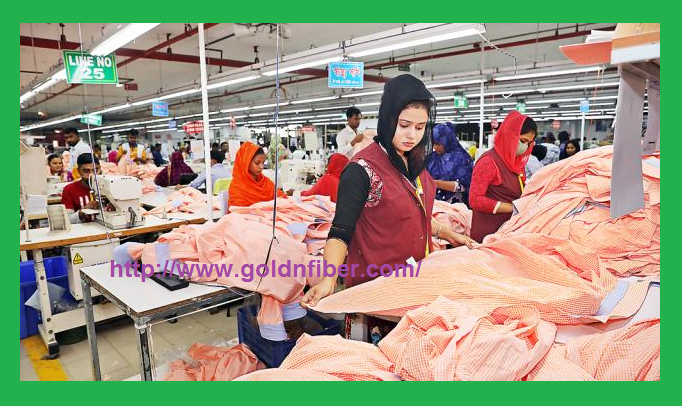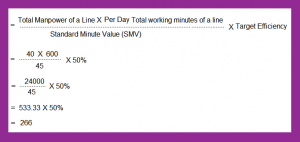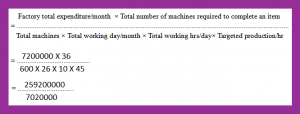## Goldnfiber | Apparel Merchandising Blog

#### Largest collection of Apparel Merchandising, Fashion Merchandising Notes, Tutorials, Tips, Experiences, Resources, Books, Jobs and OthersIntroduction:

The world is changing rapidly and human fashion trend becomes clumsy day by day.  Globalization makes product development process very tough. Thus, the apparel product developer has to involve directly through the entire process from concept to delivery. Since the product developer works in cycle with the merchandiser, having a strong background in forecasting and mathematical skills is a plus. Many garments in Bangladesh have to work on CM basis. If someone doesn’t calculate cost of making (CM), how can they fix product pricing? Thus, they should know cost of making (CM) promptly. Every garments merchandiser should know the CM calculating method. Analyze of CM i.e. cost of making for any garment items is an important part in garments merchandising. If it is not done properly then factory may fall in deep trouble. That will incur a big loss to the factory. Industrial Engineering Department can play a very important role in this regard. Today I will try to deliver some simple but effective easy methods for calculating cost of making (CM) of any garment item considering the importance of CM in garments merchandising.

#### How to Calculate Cost of Making (CM) of Garments?

Today, I will share three easy options to calculate cost of making (CM). You may follow these to enrich your skill.

A garment merchandiser should find out and consider the following parameters before going to analyzing cost of making (CM) for any garment items. But he who will try to find out CM should have some prior data to make it accurate. He should know factory approximate per month cost, approximate per line cost, SMV & item wise production process clearly:

1. Should calculate factory total expenses and overhead cost per month (running), it includes factory rent, transportation cost, utility bill, commercial charge, worker & staff wages, entertainment cost etc.
2. Count Total number of machines in the factory.
3. Count Total number of machines required in the line to complete that particular item.
4. Estimated production output per hour from the existing layout (excluding alter and reject).
5. Consider Total working day per month.
6. Consider Total working hours per day.
7. Number of manpower employed in a line
8. Sound idea of Standard minute value (SMV)
9. Estimated efficiency to achieve the target

Now we will discuss how to fix CM through SAM. It would be better if CM fix before taking any bulk order.

To find out CM (Cost of Making) in a scientific way we can follow the following path:

1. First should count SAM of the Garment
2. 2. Should count average line efficiency
3. 3. Calculate Minute cost of the labor

Now a garment merchandiser can easily find out per piece actual garment making cost by applying the following formula.

1st Option: Equation of Cost of making (CM) per pcs,

Now we will deliver an example to realize the gist point of the above discussion.

Guess,

RM leather bags and luggage factory ltd. has received an order of 5,000 pieces particular style backpack item.

Where,
Total number of manpower in a line-40

Total number of minutes to complete the item-45

Per day total number of minutes working time of a particular line-600

Estimated efficiency target-50%

Per line each day estimated average operating cost- \$500

Now we will find out cost of making (CM) of the mentioned item by the following equations.

#### Explanation:

Just input the figures, you will get cost of making (CM) per pieces backpack very soon.= \$500 / 266

= \$1.87 each backpack
So, the cost of making (CM) per backpack is about \$1.87 by using 1st formula

2nd Option: Equation of Cost of making (CM) per pcs,
Now, we will deliver an example to realize the gist point of the above discussion.

Guess,
RK leather bags and luggage factory ltd. has received an order of 5,000 pieces particular style backpack item.

Where,
Rider leather bags and luggage factory ltd. total expenses per month is about- 72,00,000 TK
Total number of machines in the factory-600
Total number of machines in a line that is necessary to complete an item-36
Estimated production output per hour from the designated layout-45
Total working day per month-26
Total working hours per day-10

Now we will find out cost of making (CM) of the mentioned item by the following equations.

#### Explanation:

Just input the figures, you will get cost of making (CM) per pieces backpack very soon.= 36.93 taka per pcs
= (36.93 × 12) taka per dozen
= 443.16 taka per dozen
= 443.16/83 (in dollar) {1dollar-83 taka (running)}
= \$5.33 per dozen

So, the cost of making (CM) per dozen of backpack is about \$5.33 by using 2nd formula

3rd Option: Equation of Cost of making (CM) per pcs,
We can get CM in another way. At first fix SAM of the individual item by examining it part by part physically to make sure that the factory is capable of doing the order in time or not. To get CM of this module we can follow the following formula:

Fix Labor cost per minute = (Monthly salary of an operator/Total minutes available in the month) at 100% efficiency
CM cost = (SAM of the garment * Minute cost of the labor)/Line efficiency (%)

Example:

If a simple backpack SMV is 20 minutes & labor cost is \$ 0.09 then what will be CM.

Here given,

SMV=20 minute

Labor cost=\$0.09

CM=SMVX Labor cost per minute

CM=20x\$0.09

CM=\$1.80 (with 100% efficiency)

But remember workers can’t achieve 100% efficiency anyway. If line efficiency becomes down then labor cost will hike automatically.

If we consider per dozen then

CM=\$1.80×12

CM=\$21.60

So, CM will be \$21.60

Conclusion:
I think these three methods what I have delivered among all is very simple but effective. Here I just tried to show the calculating method only through some fake figures. All the numbers are used here just only to meet the equations, nothing else. You have to input your actual figures to get the optimum result.